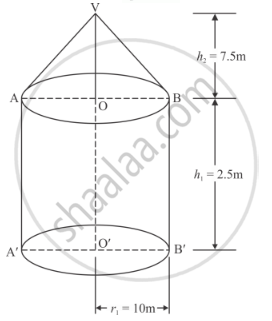Share

# A Tent is in the Form of a Cylinder of Diameter 20 M and Height 2.5 M, Surmounted by a Cone of Equal Base and Height 7.5 M. Find the Capacity of the Tent and the Cost of the Canvas at Rs 100 per Square Metre. - CBSE Class 10 - Mathematics

ConceptSurface Areas and Volumes Examples and Solutions

#### Question

A tent is in the form of a cylinder of diameter 20 m and height 2.5 m, surmounted by a cone of equal base and height 7.5 m. Find the capacity of the tent and the cost of the canvas at Rs 100 per square metre.

#### Solution

Given that:

Radius of the base r=d/2=20/2=10 m

Height of the cylinder h1 = 2.5 m

Height of the cone h2 = 7.5 mSlant height of the cone

l = sqrt(r^2+h^2)

=sqrt(10^2+7.5^2)

= 12.5 m

The total capacity of the tent is given by

V=pir^2h_1+1/3pir^2h_2

=rxx10^2xx2.5+1/3xxpixx10^2xx7.5

= π x 250 + π x 250

= 500π m3

The total area of canvas required for the tent is

S = 2πrh + πrl

= 2 x 3.14 x 10 x 2.5 + 3.14 x 10 x 12.5

= π(2 x 10 x 2.5 + 10 x 12.5)

= π(50 + 125)

= 22/7xx175

= 550 m2

Therefore, the total cost of the canvas is

= 100 x 550

= Rs. 55000

Hence, the total capacity and cost is V = 500π m3, and Rs. 55000

Is there an error in this question or solution?

#### APPEARS IN

RD Sharma Solution for 10 Mathematics (2018 to Current)
Chapter 14: Surface Areas and Volumes
Ex. 14.20 | Q: 11 | Page no. 61

#### Video TutorialsVIEW ALL 

Solution A Tent is in the Form of a Cylinder of Diameter 20 M and Height 2.5 M, Surmounted by a Cone of Equal Base and Height 7.5 M. Find the Capacity of the Tent and the Cost of the Canvas at Rs 100 per Square Metre. Concept: Surface Areas and Volumes Examples and Solutions.
S